# Creating Partial Curvilinear Length DimensionsThis task will show you how to create dimensions for the curvilinear length of a curve portion, i.e. to measure the partial length of a curve.

Partial curvilinear length dimensions are defined using points. You can use two different methods:

## Creating partial curvilinear length dimensions using existing points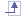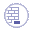Open the CurvilinearDimension.CATDrawing document. Create two points on a curve, for example. These points will be used to define the extremities of the curve portion to dimension.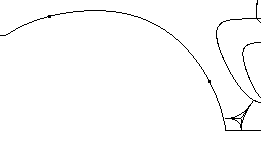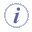You can also use spline control points (but there is none in the sample provided for this scenario), or points created in free space. In the case of points in free space, the partial curvilinear length dimension will be computed according to the normal projection of these points on the curve. So, when creating such points, you need to make sure that they will be projected on the curve, as shown below for example.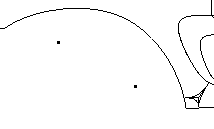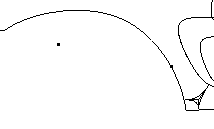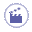1. Click Dimension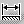in the Dimensioning toolbar.

1. Select the curve on which you created the points. A preview of the dimension is displayed. By default, this preview shows an overall curve dimension.

2. Right-click to display the contextual menu and select Partial Curvilinear Length instead of Overall.

1. Still in the contextual menu, select a representation mode for the dimension line:

• Offset displays the dimension line as an offset of the measured curve.

• Parallel displays the dimension line as a translation of the measured curve.

• Linear displays the dimension line as linear.

For the purpose of this scenario, select Parallel.

2. On the curve, select the existing point that defines the first extremity of the curve portion to dimension.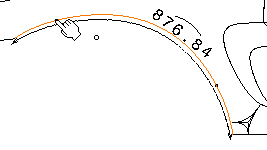3. Select the point that defines the second extremity of the curve portion to dimension.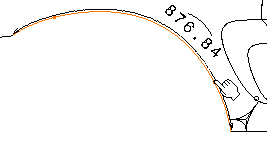4. Optionally drag the dimension line and/or the dimension value to position them as wanted.

5. Click elsewhere in the drawing to validate the dimension creation. The semi-arc symbol displayed over the dimension value symbolizes a curvilinear length dimension (whether partial or not). You can now handle the dimension just like any other dimension.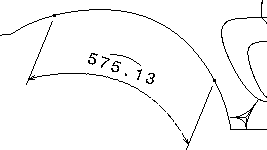6. Move one or both points, on the line or in free space. The dimension is re-computed (if you moved the point in free space, it is re-computed according to the normal projection of the points on the curve).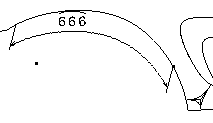If you move a point in such a way that it cannot be projected on the curve anymore, the dimension becomes not-up-to-date.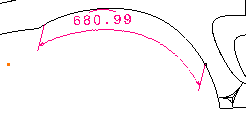## Creating partial curvilinear length dimensions using points created on-the-flyUse the CurvilinearDimension.CATDrawing document from the previous scenario, but delete the points and the dimension you created previously.1. Click Dimensionin the Dimensioning toolbar.

1. Select a curve. A preview of the dimension is displayed.

1. Right-click to display the contextual menu and make sure Partial Curvilinear Length is selected.

2. Still in the contextual menu, select a representation mode for the dimension line: for the purpose of this scenario, select Offset.

3. Select the first extremity of the curve portion to dimension by clicking in the free space, which is represented by the green projection point.
Note that the indicated point cannot go further than the extremity of the curve itself.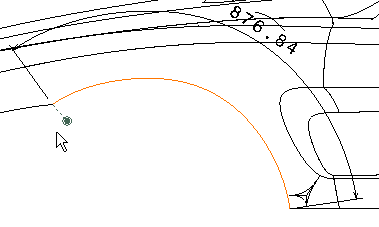4. Select the point that defines the second extremity of the curve portion to dimension in the similar way.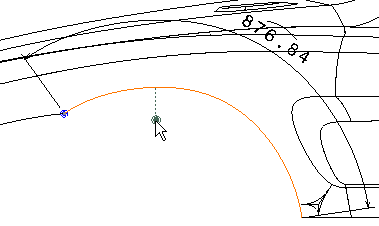Note that two points, as well as two coincidence constraints, have been created on the curve, at the projection point of where you clicked.

5. Optionally drag the dimension line and/or the dimension value to position them as wanted.

6. Click elsewhere in the drawing to validate the dimension creation. The semi-arc symbol displayed over the dimension value symbolizes a curvilinear length dimension (whether partial or not). You can now handle the dimension just like any other dimension.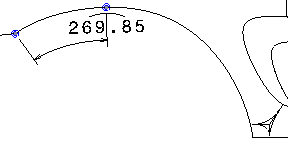## More About Partial Curvilinear Length Dimensions

### General remarks

• Partial curvilinear length dimensions can be created using the Dimensions and Length/Distance Dimensions commands; they cannot be created using the Stacked Dimensions and Cumulated Dimensions commands.
• You can create partial curvilinear length dimensions for all types of curves: splines, circles, arcs of circle, conics, etc. Note that in the case of circles and arcs of circle, they will be called partial circular length dimensions.
• You can create partial length dimensions for lines.
• The curvilinear length symbol is defined by the administrator in the standards. The same symbol is used for partial curvilinear dimensions and for curvilinear dimensions.
• Partial curvilinear length dimensions cannot be True Length dimensions.
• If you delete a point that defines a dimension, the dimension becomes not-up-to-date, and its color changes to fuchsia by default (or according to the color defined for Not-up-to-date dimensions in the Types and colors of dimensions dialog box available via Tools > Options > Mechanical Design > Drafting > Dimension tab, Analysis Display Mode area, Types and colors... button). If you delete both points, the dimension becomes a regular curvilinear dimension.

### Restrictions

• In the case of the parallel and offset representation modes, the dimension value cannot be moved out of the curve limits, except for circles and arcs of circle. As a result, you cannot specify the dimension value position (Inside, Outside, Auto).
• In some cases, depending on the curve and on the offset value, the offset representation mode cannot be computed.
• In the case of partial curvilinear length dimensions in offset mode, it is recommended to activate the Constant offset between dimension line and geometry setting in Tools > Options > Mechanical Design > Drafting > Dimension tab. This will ensure that the dimension remains associative if the geometry is moved.
• Partial curvilinear dimensions cannot be measured along a direction. However, partial length dimensions can be measured along a direction.
• Partial curvilinear dimensions cannot be driving dimensions.
• When creating partial circular length dimensions on circles, you cannot select a circular sector.